Scan QR code or get instant email to install app

Question:

# 1212Solve: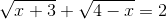A no solution
explanation

1212Since solving by substitution is required to confirm your answer, and is the simpler method, this answer could certainly be found by plugging in the given values. However, since all tests are not multiple choice and you really need to understand the total process, here it is: Begin by isolating a square root: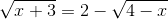, square both sides: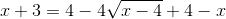, combine like terms: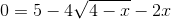, isolate the square root: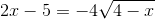, square both sides: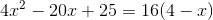, rewrite as a quadratic: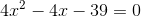, use quadratic formula to find: x = 3.66 and x = -2.66. It is necessary to substitute these values back into the original expression to test their validity: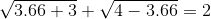, which becomes: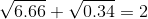, which is not true, so this x value is invalid. Testing the other value: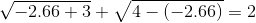, which becomes: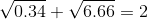, which is not true, so this x value is also invalid. There are no solutions.